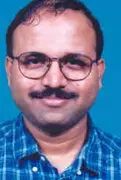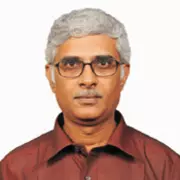X
Ultrasonic band gaps in periodic stack of plates - Simulation and experiments
B. C. Ram, Kumar P. Padma,
Published in
2006
Volume: 820 II

Pages: 1043 - 1050
Abstract
Ultrasonic band gaps were simulated using various numerical techniques for 1-D periodic structures. The pulse propagation characteristics were simulated using transient finite element analysis. The frequency domain characteristics were simulated using forced periodic response analysis, Thompson Haskell transfer matrix and acoustic impedance based calculations. Dispersion curves were also obtained using eigen value lattice simulations. Simulation of the band gaps obtained using these numerical methods agreed well with each other. Experiments were carried out on layered material (aluminum-plexiglas) at ultrasonic frequencies. The measured band gaps matched with the simulation results. Numerical and experimental results are also presented for mirror symmetric periodic stacks. © 2006 American Institute of Physics.
••